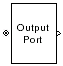# Output Port

Connection block from RF physical blocks to Simulink environment

•Libraries:
RF Blockset / Equivalent Baseband / Input / Output Ports

## Description

The Output Port block produces the baseband-equivalent time-domain response of an input signal traveling through a series of RF physical components. The Output Port block

1. Partitions the RF physical components into linear and nonlinear subsystems.

2. Extracts the complex impulse response of the linear subsystem for baseband-equivalent modeling of the RF linear system.

3. Extracts the nonlinear AMAM/AMPM modeling for RF nonlinearity.

The Output Port block also serves as a connecting port from an RF physical part of the model to the Simulink®, or mathematical, part of the model. For more information about how the Output Port block converts the physical modeling environment signals to mathematical Simulink signals, see Convert to and from Simulink Signals.

Note

Some RF blocks require the sample time to perform baseband modeling calculations. To ensure the accuracy of these calculations, the Input Port block, as well as the mathematical RF blocks, compare the input sample time to the sample time you provide in the mask. If they do not match, or if the input sample time is missing because the blocks are not connected, an error message appears.

## Parameters

expand all

### Main Tab

Load impedance of the RF network described in the physical model to which it connects, specified as scalar in ohms.

### Visualization Tab

Frequency data source in specified based on one of the following:

• When Source of frequency data is ```Derived from Input Port parameter```, frequency data source will be derived from the parameters set on the Input Port.

• When Source of frequency data is `User-specified`, specify as a vector of frequencies in the Frequency data parameter.

Frequency data range, specified as a vector in hertz.

#### Dependencies

To enable this parameter, set `User-specified` in Source of amplifier gain.

Reference impedance of the coaxial transmission line, specified as a scalar in ohms.

Type of data plot that you want to produce with your data specified as:

• `X-Y plane` — Generate a Cartesian plot of your data versus frequency. To create linear, semi-log, or log-log plots, set the Y scale and X scale accordingly.

• ```Composite data```—The composite data plot automatically generates four separate plots in one figure window, showing the frequency dependence of several parameters.

• `Polar plane` — Generate a polar plot of your data. The block plots only the range of data corresponding to the specified frequencies.

• `Z Smith chart`, `Y Smith chart`, and `ZY Smith chart` — Generate a Smith® chart of your data. The block plots only the range of data corresponding to the specified frequencies.

Type of parameters to plot based on the Plot type you set, specified as one of the following.

Plot typeY parameter1
`X-Y plane``S11`, `S12`, `S21`, `S22`, `Gt`, `GroupDelay`, `GammaIn`, `GammaOut`, `VSWRIn`, `VSWROut`, `OIP3`, `NF`, `NFactor`, and `NTemp`.
`Composite data`No Y parameter1 to set.
```Polar plane````S11`, `S12`, `S21`, `S22`, `GammaIn`, and `GammaOut`
`Z Smith chart``S11`, `S22`, `GammaIn`, and `GammaOut`.
`Y Smith chart``S11`, `S22`, `GammaIn`, and `GammaOut`.
```ZY smith chart````S11`, `S22`, `GammaIn`, and `GammaOut`.

Type of parameters to plot based on the Plot type you set, specified as one of the following.

Plot typeY parameter2
`X-Y plane``S11`, `S12`, `S21`, `S22`, `Gt`, `GroupDelay`, `GammaIn`, `GammaOut`, `VSWRIn`, `VSWROut`, `OIP3`, `NF`, `NFactor`, and `NTemp`.
`Composite data`No Y parameter2 to set.
```Polar plane````S11`, `S12`, `S21`, `S22`, `GammaIn`, and `GammaOut`
`Z Smith chart``S11`, `S22`, `GammaIn`, and `GammaOut`.
`Y Smith chart``S11`, `S22`, `GammaIn`, and `GammaOut`.
```ZY smith chart````S11`, `S22`, `GammaIn`, and `GammaOut`.

Plot format, specified as one of the following.

Y prarameter1Y format1
`S11`, `S12`, `S21`, `S22`, `GammaIn`, and `GammaOut`.`dB`, `Magnitude (decibels)`, `Abs`, `Mag`, `Magnitude (linear)`, `Angle`, `Angle(degrees)`, `Angle(radians)`, `Real`, `Imag`, and `Imaginary`.
`GroupDelay``ns`, `us`, `ms`, `s`, and `ps`.
`VSWRIn`, `VSWROut`, and `Gt`.```Magnitude (decibels)``` and `None`.
`OIP3``dBm`, `dBW`, `W`, and `mW`.
`NF` ```Magnitude (decibels)```.
`NFactor``None`
`NTemp``Kelvin`

#### Dependencies

To enable Y format1, set Plot type to `X-Y plane`.

Plot format, specified as one of the following.

Y prarameter2Y format2
`S11`, `S12`, `S21`, `S22`, `GammaIn`, and `GammaOut`.`dB`, `Magnitude (decibels)`, `Abs`, `Mag`, `Magnitude (linear)`, `Angle`, `Angle(degrees)`, `Angle(radians)`, `Real`, `Imag`, and `Imaginary`.
`GroupDelay``ns`, `us`, `ms`, `s`, and `ps`.
`VSWRIn`, `VSWROut`, and `Gt`.```Magnitude (decibels)``` and `None`.
`OIP3``dBm`, `dBW`, `W`, and `mW`.
`NF` ```Magnitude (decibels)```.
`NFactor``None`
`NTemp``Kelvin`

#### Dependencies

To enable Y format2, set Plot type to `X-Y plane`.

Parameter, specified as `Freq`. This parameter determines the data for x-axes on the X-Y plane plot.

Plot format, specified as one of the following `Hz`, `Auto`, `KHz`, `MHz`, `GHz` or `THz`.

Y-axis scale, specified as `Linear` or `Log`.

X-axis scale, specified as `Linear` or `Log`.

Plot the specified data using the plot button.

Dependencies

The Visualization tab shows parameters for creating plots if you display the Output Port mask after you perform one or more of the following actions:

• Run a model with two or more blocks between the Input Port block and the Output Port block.

• Click the Update Diagram button to initialize a model with two or more blocks between the Input Port block and the Output Port block.

For information about plotting, see Create Plots Using Equivalent Baseband Library Blocks.